Next: Ideal monatomic gases Up: Applications of statistical thermodynamics Previous: Mean values

## Partition functions

It is clear that all important macroscopic quantities associated with a system can be expressed in terms of its partition function. Let us investigate how the partition function is related to thermodynamical quantities. Recall thatis a function of both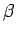and(whereis the single external parameter). Hence,, and we can write(415)

Consider a quasi-static change by whichandchange so slowly that the system stays close to equilibrium, and, thus, remains distributed according to the Boltzmann distribution. If follows from Eqs. (399) and (409) that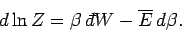(416)

The last term can be rewritten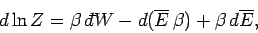(417)

giving(418)

The above equation shows that although the heat absorbed by the systemis not an exact differential, it becomes one when multiplied by the temperature parameter. This is essentially the second law of thermodynamics. In fact, we know that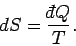(419)

Hence,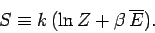(420)

This expression enables us to calculate the entropy of a system from its partition function.

Suppose that we are dealing with a system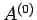consisting of two systemsandwhich only interact weakly with one another. Let each state ofbe denoted by an indexand have a corresponding energy. Likewise, let each state ofbe denoted by an index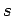and have a corresponding energy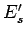. A state of the combined systemis then denoted by two indicesand. Sinceandonly interact weakly their energies are additive, and the energy of state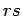is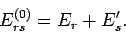(421)

By definition, the partition function oftakes the form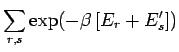(422)

Hence,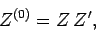(423)

giving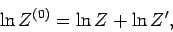(424)

whereand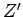are the partition functions ofand, respectively. It follows from Eq. (399) that the mean energies of,, andare related by(425)

It also follows from Eq. (420) that the respective entropies of these systems are related via(426)

Hence, the partition function tells us that the extensive thermodynamic functions of two weakly interacting systems are simply additive.

It is clear that we can perform statistical thermodynamical calculations using the partition functioninstead of the more direct approach in which we use the density of states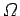. The former approach is advantageous because the partition function is an unrestricted sum of Boltzmann factors over all accessible states, irrespective of their energy, whereas the density of states is a restricted sum over all states whose energies lie in some narrow range. In general, it is far easier to perform an unrestricted sum than a restricted sum. Thus, it is generally easier to derive statistical thermodynamical results usingrather than, althoughhas a far more direct physical significance than.Next: Ideal monatomic gases Up: Applications of statistical thermodynamics Previous: Mean values
Richard Fitzpatrick 2006-02-02